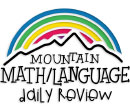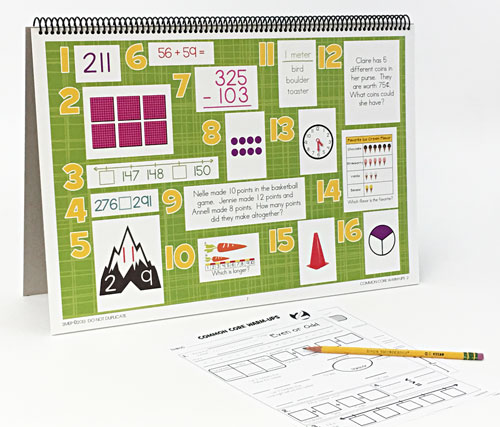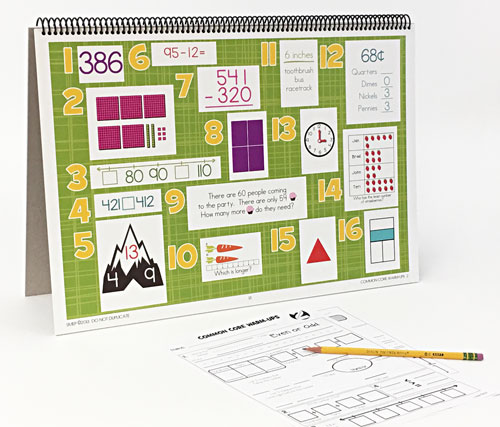WHAT YOU GET:
• 32 WEEKS OF DAILY REVIEW
• INSTRUCTIONS
• ONE MASTER STUDENT RESPONSE SHEET
• HEAVY DUTY STAND
• KNOWLEDGEABLE STAFF FOR ANY ASSISTANCE

This 2nd GRADE COMMON CORE DAILY REVIEW MATH CENTER EDITION is the DAILY REVIEW your students need to MASTER the concepts you have taught.

Research has proven that LONG-TERM MASTERY comes from SPIRAL REVIEW, also called DISTRIBUTED PRACTICE AND SPACED REPETITION.

The CENTER EDITION is designed for small group review, such as rotations, scoots, centers, or math-around-the-room. It is a smaller version (13"x19") of our Bulletin Board Kit. It can easily be set up and taken down as needed.
1. 2.NBT.A.1.A The numbers 100, 200, 300, 400, 500, 600, 700, 800, 900 refer to one, two, three, four, five, six, seven, eight, or nine hundreds (and 0 tens and 0 ones).
2.NBT.A.3 Read and write numbers to 1000 using base-ten numerals, number names, and expanded form.
2.NBT.B.8 Mentally add 10 or 100 to a given number 100-900, and mentally subtract 10 or 100 from a given number 100-900.
2.OA.C.3 Determine whether a group of objects (up to 20) has an odd or even number of members, e.g., by pairing objects or counting them by 2s; write an equation to express an even number as a sum of two equal addends.
2. 2.NBT.A.1.A The numbers 100, 200, 300, 400, 500, 600, 700, 800, 900 refer to one, two, three, four, five, six, seven, eight, or nine hundreds (and 0 tens and 0 ones).
2.NBT.A.3 Read and write numbers to 1000 using base-ten numerals, number names, and expanded form.
3. 2.NBT.A.2 Count within 1000; skip-count by 5s, 10s, and 100s.
4. 2.NBT.A.4 Compare two three-digit numbers based on meanings of the hundreds, tens, and ones digits, using >, =, and < symbols to record the results of comparisons.
5. 2.OA.B.2 Fluently add and subtract within 20 using mental strategies. By end of Grade 2, know from memory all sums of two one-digit numbers.
6. 2.NBT.B.5 Fluently add and subtract within 100 using strategies based on place value, properties of operations, and/or the relationship between addition and subtraction.
2.NBT.B.6 Add up to four two-digit numbers using strategies based on place value and properties of operations.
7. 2.NBT.B.7 Add and subtract within 1000, using concrete models or drawings and strategies based on place value, properties of operations, and/or the relationship between addition and subtraction; relate the strategy to a written method. Understand that in adding or subtracting three-digit numbers, one adds or subtracts hundreds and hundreds, tens and tens, ones and ones; and sometimes it is necessary to compose or decompose tens or hundreds.
8. 2.OA.C.3 Determine whether a group of objects (up to 20) has an odd or even number of members, e.g., by pairing objects or counting them by 2s; write an equation to express an even number as a sum of two equal addends.
2.OA.C.4 Use addition to find the total number of objects arranged in rectangular arrays with up to 5 rows and up to 5 columns; write an equation to express the total as a sum of equal addends.
2.G.A.2 Partition a rectangle into rows and columns of same-size squares and count to find the total number of them.
9. 2.OA.A.1 Use addition and subtraction within 100 to solve one- and two-step word problems involving situations of adding to, taking from, putting together, taking apart, and comparing, with unknowns in all positions, e.g., by using drawings and equations with a symbol for the unknown number to represent the problem.
2.NBT.B.9 Explain why addition and subtraction strategies work, using place value and the properties of operations.
2.MD.B.5 Use addition and subtraction within 100 to solve word problems involving lengths that are given in the same units, e.g., by using drawings (such as drawings of rulers) and equations with a symbol for the unknown number to represent the problem.
2.MD.B.6 Represent whole numbers as lengths from 0 on a number line diagram with equally spaced points corresponding to the numbers 0, 1, 2, ..., and represent whole-number sums and differences within 100 on a number line diagram.
10. 2.MD.A.1 Measure the length of an object by selecting and using appropriate tools such as rulers, yardsticks, meter sticks, and measuring tapes.
2.MD.A.4 Measure to determine how much longer one object is than another, expressing the length difference in terms of a standard length unit.
11. 2.MD.A.3 Estimate lengths using units of inches, feet, centimeters, and meters.
12. 2.MD.C.8 Solve word problems involving dollar bills, quarters, dimes, nickels, and pennies, using \$ and ¢ symbols appropriately.
13. 2.MD.C.7 Tell and write time from analog and digital clocks to the nearest five minutes, using a.m. and p.m.
14. 2.MD.D.9 Generate measurement data by measuring lengths of several objects to the nearest whole unit, or by making repeated measurements of the same object. Show the measurements by making a line plot, where the horizontal scale is marked off in whole-number units.
2.MD.D.10 Draw a picture graph and a bar graph (with single-unit scale) to represent a data set with up to four categories. Solve simple put-together, take-apart, and compare problems1 using information presented in a bar graph.
15. 2.G.A.1 Recognize and draw shapes having specified attributes, such as a given number of angles or a given number of equal faces. Identify triangles, quadrilaterals, pentagons, hexagons, and cubes.
16. 2.G.A.3 Partition circles and rectangles into two, three, or four equal shares, describe the shares using the words halves, thirds, half of, a third of, etc., and describe the whole as two halves, three thirds, four fourths. Recognize that equal shares of identical wholes need not have the same shape.
ONLINE REVIEW VS.
CENTER EDITION VS.
BULLETIN BOARD KIT
ONLINE REVIEW
Is projected onto an interactive white board, creating a virtual board. It can be viewed by the entire classroom. Answers can be displayed onto the board at the click of a button. Up to seven questions can be isolated and magnified.

CENTER EDITION
Is organized on a 13"x19" flip chart. It can be viewed by 5-6 students at a time. It was designed for small group review, such as rotations, scoots, centers, or math-around-the-classroom. It has an answer key, heavy-duty stand, and high-gauged coil.

BULLETIN BOARD KIT
It is designed for total classroom view. It hangs on bulletin boards, walls, white boards, pocket charts, and science display boards. Once hung, it can remain displayed for the entire year. The cards can be interchanged, creating flexible and adjustable DAILY REVIEW.
\$95.95 QTY:
Centers   |   2nd Grade Common Core Math